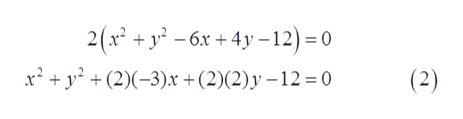# Given this equation of a circle, 2x2+ 2y2 − 12x + 8y − 24 = 0Determine the coordinates of the centerDetermine the radiusSketch the graph, labeling all intercepts

Question
4 views

Given this equation of a circle, 2x2+ 2y2 − 12x + 8y − 24 = 0

1. Determine the coordinates of the center
3. Sketch the graph, labeling all intercepts
check_circle

Step 1

The given equation of circle is

Step 2

To find:

1. The coordinates of the center of the circle.
2. The radius of the circle.
3. The sketch (graph) of the circle representing x and y intercepts.
Step 3

From (1), the equation of the c...help_outlineImage Transcriptionclose2(x* + y - 6x + 4y – 12) = 0 x² + y° + (2)(-3)x +(2)(2)y –12=0 (2) fullscreen

### Want to see the full answer?

See Solution

#### Want to see this answer and more?

Solutions are written by subject experts who are available 24/7. Questions are typically answered within 1 hour.*

See Solution
*Response times may vary by subject and question.
Tagged in

### Algebra